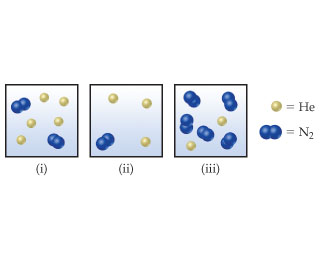# Problem: Consider the following samples of gases:If the three samples are all at the same temperature, rank them with respect to partial pressure of helium (PHe).

###### FREE Expert Solution

Recall that the partial pressure of a gas (PGas) in a mixture is given by:

$\overline{){{\mathbf{P}}}_{{\mathbf{gas}}}{\mathbf{=}}{{\mathbf{\chi }}}_{{\mathbf{gas}}}{{\mathbf{P}}}_{{\mathbf{total}}}}$

where:

χGas = mole fraction of the gas

Ptotal = total pressure of the gas mixture###### Problem Details

Consider the following samples of gases:If the three samples are all at the same temperature, rank them with respect to partial pressure of helium (PHe).